# Blog

Analysis of a Function with Derivatives using UMS

# 部落格

Analysis of a Function with Derivatives using UMS

# Blog

Analysis of a Function with Derivatives using UMS

# Блог

Analysis of a Function with Derivatives using UMS

# Blog

Analysis of a Function with Derivatives using UMS

# Blog

Analysis of a Function with Derivatives using UMS

# בלוג

Analysis of a Function with Derivatives using UMS

# Blogue

Analysis of a Function with Derivatives using UMS

# Blog

Analysis of a Function with Derivatives using UMS

# Blog

Analysis of a Function with Derivatives using UMS

## Analysis of a Function with Derivatives using UMS

Maxima and minima, Fermat’s theorem, critical points, first derivative test.
In mathematics, the maximum and minimum (plural: maxima and minima) of a function, known collectively as extrema (singular: extremum), are the largest and smallest value that the function takes at a point either within a given neighborhood (local or relative extremum) or on the function domain in its entirety (global or absolute extremum)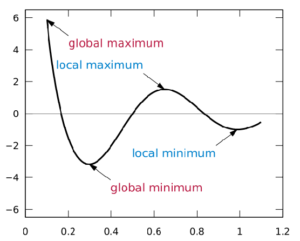A real-valued function f defined on a domain X  has a global (or absolutemaximum point at x* if f (x* ) ≥ f(x) for all  in . Similarly, the function has a global (or absoluteminimum point at x* if f (x* ) ≤ f(x) for all  x in X. The value of the function at a maximum point is called the maximum value of the function and the value of the function at a minimum point is called the minimum value of the function.

Finding functional maxima and minima
Finding global maxima and minima is the goal of mathematical optimization. If a function is continuous on a closed interval, then by the extreme alue theorem global maxima and minima exist. Furthermore, a global maximum (or minimum) either must be a local maximum (or minimum) in the interior of the domain, or must lie on the boundary of the domain. So a method of finding a global maximum (or minimum) is to look at all the local maxima (or minima) in the interior, and also look at the maxima (or minima) of the points on the boundary, and take the largest (or smallest) one.Local extrema of differentiable functions can be found by Fermat’s theorem, which states that they must occur at critical points. One can distinguish whether a critical point is a local maximum or local minimum by using the first derivative test, second derivative test, or higher-order derivative test, given sufficient differentiability.For any function that is defined piecewise, one finds a maximum (or minimum) by finding the maximum (or minimum) of each piece separately, and then seeing which one is largest (or smallest).

Fermat’s theorem ( interior extremum theorem )
In mathematics, Fermat’s theorem (also known as Interior extremum theorem) is a method to find local maxima and minima of differentiable functions on open sets by showing that every local extremum of the function is a stationary point (the function derivative is zero in that point). Fermat’s theorem is a theorem in real analysis, named after Pierre de Fermat.By using Fermat’s theorem, the potential extrema of a function f with derivative  f , are found by solving an equation in  f ‘. Fermat’s theorem gives only a necessary condition for extreme function values, and some stationary points are inflection points (not a maximum or minimum). The function’s second derivative, if it exists, can determine if any stationary point is a maximum, minimum, or inflection point.

Fermat’s theorem
One way to state Fermat’s theorem is that whenever you compute the derivative of a function’s local extrema, the result will always be zero. In precise mathematical language: Let
f:(a,b) →R be a function and suppose that x0 in (a,b)  is a local extremum of f .
If f is differentiable at x0  then f ‘(x0)=0 . Another way to understand the theorem is via the contrapositive statement. If the derivative of a function at any point is not zero, that point is not an extrema. Formally: If f is differentiable at x0 in (a,b) , and f ‘(x0)≠0 , then  x0 is not a local extremum of f  .

Corollary
The global extrema of a function f on a domain  A  occur only at boundaries, non-differentiable points, and stationary points. If x0 is a global extremum of  f, then one of the following is true:
boundary
x0 is in the boundary of A
non-differentiable
f is not differentiable at  x0
stationary point
: x0  is a stationary point of  f

Critical points
In mathematics, a critical point or stationary point of a differentiable function of a real orcomplex variable is any value in its domain where its derivative is 0 or undefined.
A critical point or stationary point of a differentiable function of a single real variable,  f (x), is a value x0 in the domain of f where its derivative is 0: f ‘(x0)=0 . A critical value is the image under f of a critical point. These concepts may be visualized through the graph of  f : at a critical point, the graph has a horizontal tangent and the derivative of the function is zero.

First derivative test
First derivative test depends on the “increasing-decreasing test”, which is itself ultimately a consequence of the mean value theorem. Suppose  f is a real-valued function of a real variable defined on some interval containing the critical point a. Further suppose that f is continuous
at  a and differentiable on some open interval containing a, except possibly at a  itself.
1) If there exists a positive number r such that for every x  in ( a-r,a] we have f ‘(x )≥0 , and for every x in [a,a+r) we have f ‘(x )≤ 0, then f has a local maximum at a
2) If there exists a positive number r such that for every x in ( a-r,a) we have f ‘(x )≤ 0, and for every x in ( a,a+r) we have  f ‘(x )≥0 , then f has a local minimum at a.
3) If there exists a positive number r  such that for every x in ( a-r, a) U ( a, a+r) we have
f ‘(x ) >0 , or if there exists a positive number  r such that for every x in
( a-r, a) U ( a, a+r) we have f ‘(x ) < 0 , then f has neither a local maximum nor a local minimum at a .
If none of the above conditions hold, then the test fails. (Such a condition is not vacuous; there are functions that satisfy none of the first three conditions.)

Questions

1) How to find local max and local min of the function y(x)=x²/(x-1) using Universal Math Solver?

To determine the local maximum (max) and local minimum (min) of any rational function
y(x)=f(x) enter this function y(x)=x²/(x-1) and perform the analysis of the function (select the option “Maximum and minimum”)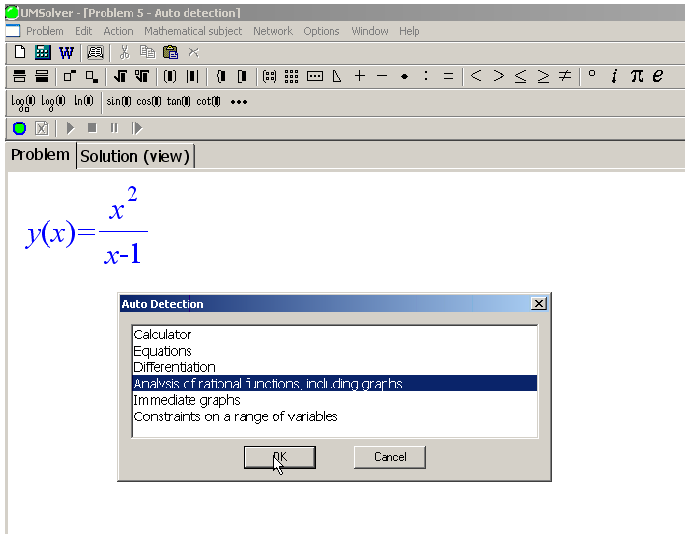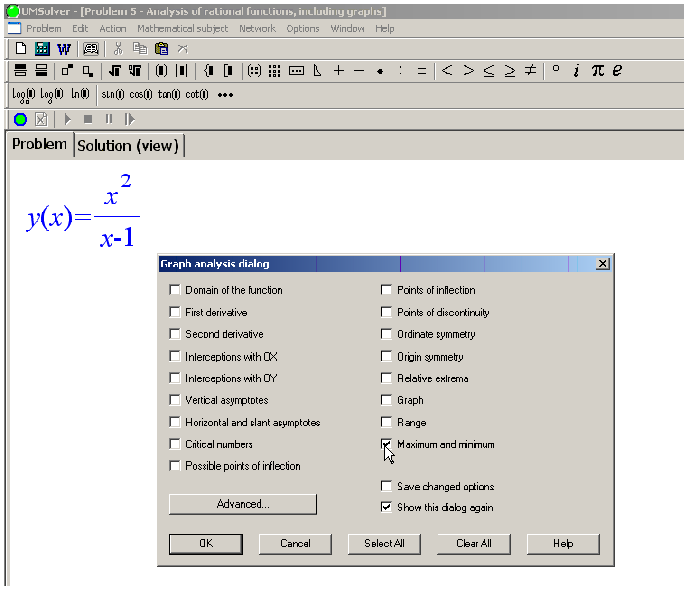UMS Solution:
Let us study the function given by: y(x)=x²/(x-1)
The domain of the function is the following set: x<1; x>1
First derivative: :  y'(x)=x(x-2)/(x-1)²
Vertical asymptotes: x=1
Critical numbers: x=0; x=2.
In order to determine critical numbers we set the first derivative equal to zero.
x(x-2)/(x-1)²=0
A fraction is equal to zero if and only if the numerator is equal to zero.
Case 1
x=0
Here is the answer to this particular case: x=0
Case 2
x-2=0
Let’s move the constants to the right−hand side of the equality with the reverse sign.
x=2
Here is the answer to this particular case: x=2
Test intervals: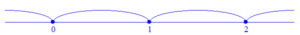The analysis of the function graph is shown in the Table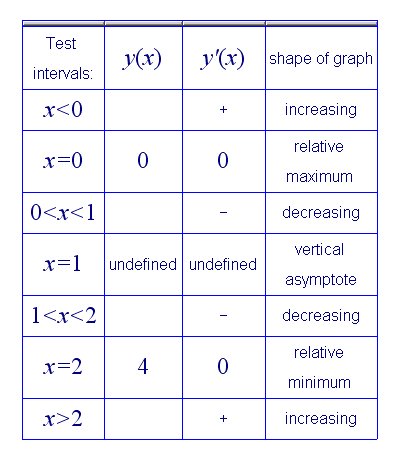The final answer is: relative minimum x=2; relative maximum x=0

2) How to find global max and global min of the function y(x)=2x³-3x²-12x-2 defined over the closed interval (segment) [-2;1] using UMS ?

To determine global max and global min of any rational function
y(x)=f(x) defined over the closed interval (segment) enter this function
y(x)=2x³-3x²-12x-2 ; -2≤x≤1 and perform the analysis of the function
(select the option “Maximum and minimum”)

UMS Solution:
Let us study the function given by: y(x)=2x³-3x²-12x-2
The domain of the function is the following set: -2≤x≤1
First derivative: y'(x)=6x²-6x-12

(2x³-3x²-12x-2)’ =
The derivative of a sum equals the sum of the derivatives.
(2x³)’ -(3x²)’-(12x)’-(2)’
The derivative of any constant equals zero.
(2x³)’ -(3x²)’-(12x)’-0=
(2x³)’ -(3x²)’-(12x)’=
The derivative of a constant times a function is equal to the constant times the derivative of the function.
2(x³)’ -3(x²)’-12(x)’=
Use the Power Rule .
2(3x²) -3(2x)-12•1=
6x² -6x-12

Vertical asymptotes: none
Critical numbers: x=-1
In order to determine critical numbers we set the first derivative equal to zero.
6x² -6x-12=0
The next equation is equivalent to the previous one
x² -x-2=0
Let us find the discriminant.
D=b² -4ac=(-1)²-4∙1∙(-2)=9
The discriminant is positive, hence the equation has two distinct real roots.
Now we use the formula for the roots of a quadratic equation
x1,2=(-b± D)/(2a)
x1=(1-3)/2=-1 ; x2=(1+3)/2=2
2 does not belong to the domain of the function.
Test intervals: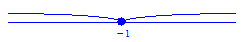The analysis of the function graph is shown in the Table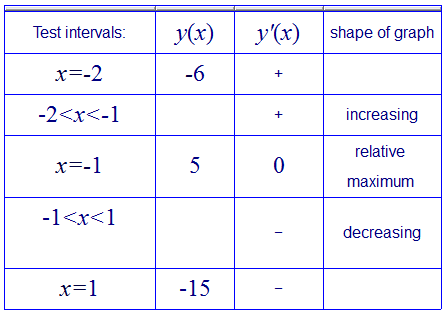Range: -15≤y≤5
Minimum:y=-15
Maximum:y=5

### 标签

For Windows OS

pour Windows

для Windows OS

voor Windows

für Windows Betriebssysteme

per Windows OS

para Windows OS

למערכת הפעלה ווינדוס

Windows İşletim Sistemi için

### פתור ותרגל שיעורי בית במתמטיקה עכשיו!

למערכת הפעלה ווינדוס

для Windows OS

### Matematik ödevinizi hemen şimdi çözün!

(Windows İşletim Sistemi için)

Pour Windows

Per Windows OS

Para Windows OS

### Löse und übe jetzt deine Mathe-Hausaufgaben!

Für Windows Betriebssysteme

Voor Windows

For Windows OS